Filter By

## All Questions

#### The ratio of maximum acceleration to maximum velocity in a simple harmonic motion is 10 s−1.  At, t=0 the displacement is 5 m.  What is the maximum acceleration ?  The initial phase ISOption: 1 500 m/s2   Option: 2  Option: 3 750 m/s2   Option: 4 m/s2

As learnt inAtMaximum acceleration =#### A particle is moving along the x- axis with its coordinate with time 't' given by . Another particle is moving along the y-axis with its coordinate as a function of time given by  At  s, the speed of the second particle measured in the frame of the first particle is given by . Then  (in m/s) is ____________. Option: 1 580 Option: 2 700 Option: 3 100 Option: 4 300

At time t= 1 s

So the value of v is 580

## Crack CUET with india's "Best Teachers"

• HD Video Lectures
• Unlimited Mock Tests
• Faculty Support#### A ball is dropped from the top of a 100 m high tower on a planet. In the last s before hitting the ground, it covers a distance of 19 m. Acceleration due to gravity ( in ms-2) near the surface on that planet is _______. Option: 1 8 Option: 2 4 Option: 3 2 Option: 4 6

Let the total time taken by the particle is t

So distance covered by a particle in t sec=

Similarly, distance covered by particle in sec=

So  distance covered by particle in last (1/2) sec of its journey=

So

and

from equations (1) and (2)

we get

putting t=5 we get g = 8 m/s2

## Crack NEET with "AI Coach"

• HD Video Lectures
• Unlimited Mock Tests
• Faculty Support#### A particle moves such that its position vector where is a constant and t is time. Then which of the following statements is true for the velocity  and acceleration  of the particle :  Option: 1andboth are parallel toOption: 2is perpendicular toandis directed away from the origin Option: 3andboth are perpendicular toOption: 4is perpendicular toandis directed towards the origin

implies a is anti parallel to r

Implies v is perpendicular to r

hence the correct option is (4).

## Crack JEE Main with "AI Coach"

• HD Video Lectures
• Unlimited Mock Tests
• Faculty Support#### The sum of two forces   and  issuch that  The angle  (in degrees) that the resultant of  and  will make with  is , Option: 1 90 Option: 2 120 Option: 3 45 Option: 4 30

As

Now Vector P is doubled but vector Q is the same so the angle between  will be also

And If resultant of  is

Then from the figure angle between  is

and

#### A car accelerates from rest at a constant ratefor sometime after which it decelerates at a constant rateto come to rest. If the total time elapsed is t seconds, the total distance travelled is :Option: 1Option: 2Option: 3Option: 4Let the car accelerate for time traveling distance and acquire maximum velocity v.
Then, [from the equation, ]
and   [from the equation, v=u+a t ]

After this car decelerates for time  to come to rest
Hence,        [from the equation, ]
and          [from the equation, u=v-a t ]

Also, total distance traveled,

## Crack CUET with india's "Best Teachers"

• HD Video Lectures
• Unlimited Mock Tests
• Faculty Support#### A swimmer wants to cross a river from pointto point. Linemakes an angle ofwith the flow of river. Magnitude of velocity of the swimmer is same as that of the river. The anglewith the lineshould be _______swimmer reaches point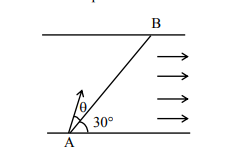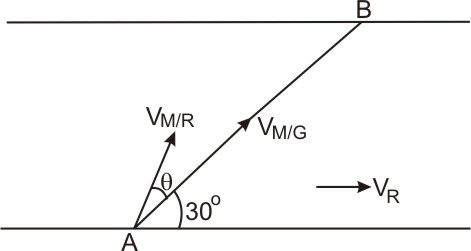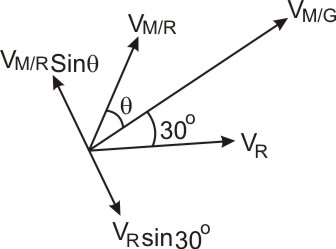#### The magnitude of vectorsin the given figure are equal. the direction ofwith x-axis will be: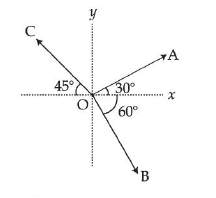Option: 1Option: 2Option: 3Option: 4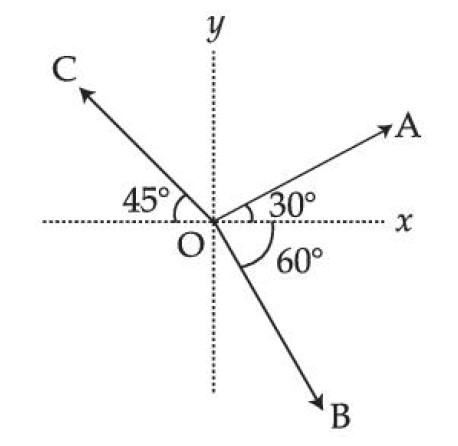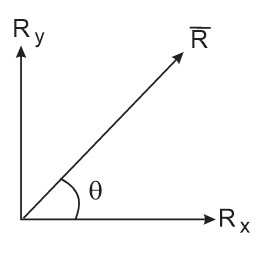The correct option is (2)